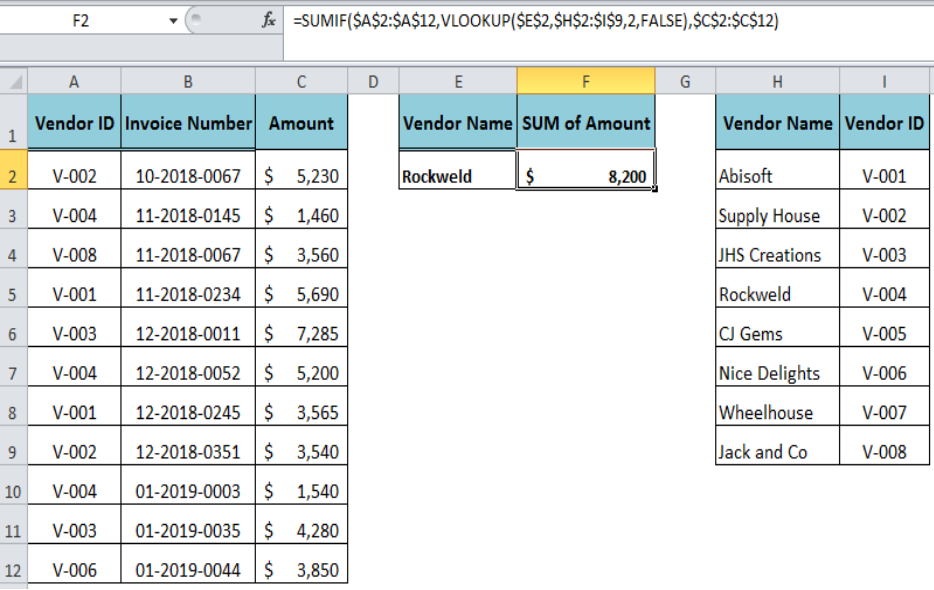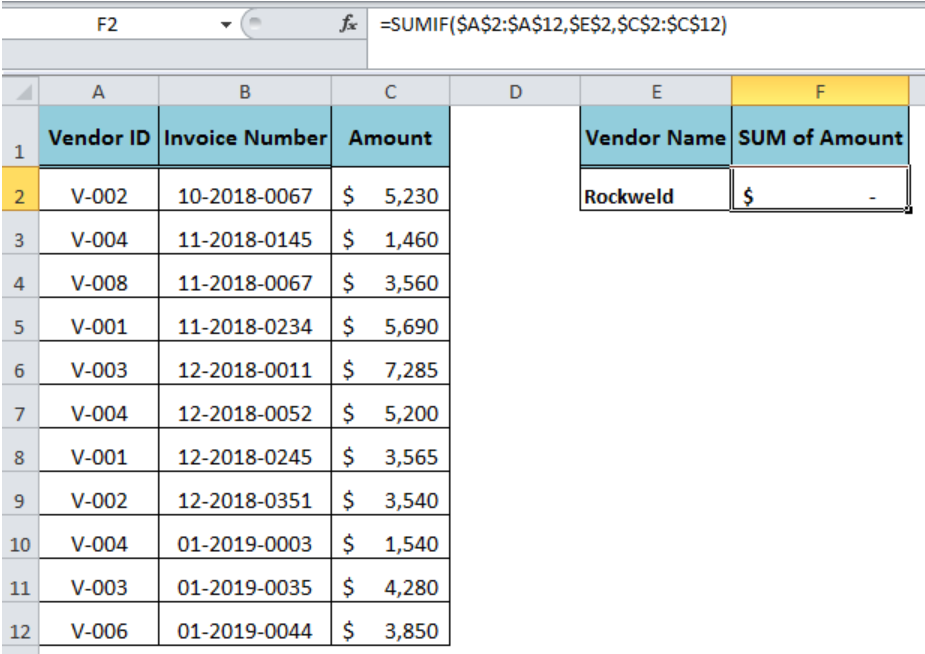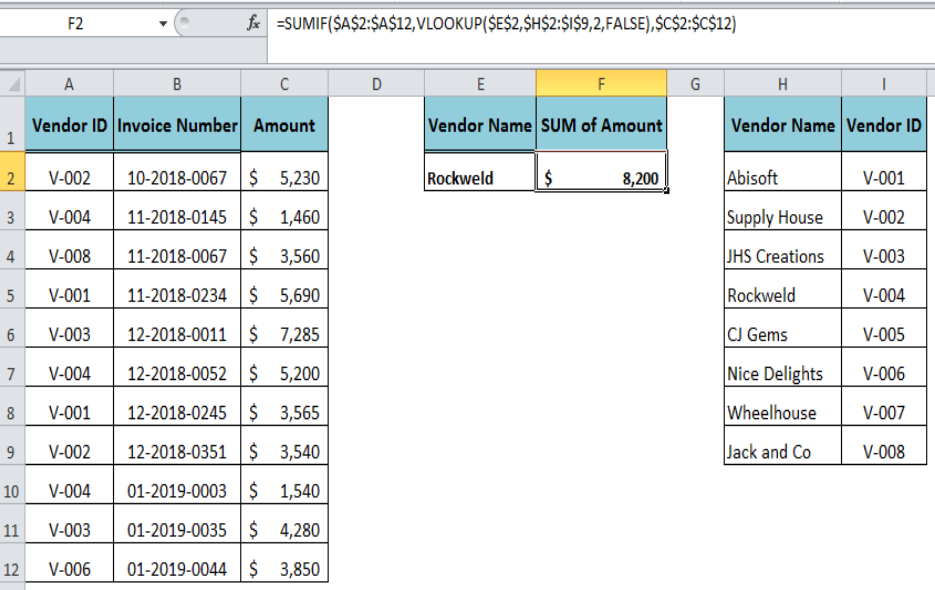Get instant live expert help with Excel or Google Sheets“My Excelchat expert helped me in less than 20 minutes, saving me what would have been 5 hours of work!”

#### Post your problem and you’ll get Expert help in seconds.

Your message must be at least 40 characters
Our professional Expert are available now. Your privacy is guaranteed.

# How to Combine the SUMIF and VLOOKUP Functions in Excel

When we need to summarize the values in a dataset based on matching instances with single criteria then we use the SUMIF function. We can also nest the VLOOKUP function inside of the SUMIF function in order to make a dynamic SUMIF function. This tutorial will step through the process.Figure 1. Combining the SUMIF and VLOOKUP Functions

## The SUMIF and VLOOKUP Functions

Suppose we have a dataset of vendors’ invoices in a table range A2:C12 where invoice numbers and amounts are listed with respect to vendors’ IDs. But we want to sum the amounts for a given “Vendor Name” as criteria. As our table range A2: C12 does not contain “Vendor Name” field, so we are unable to sum the invoices amounts using simply the SUMIF function.Figure 2. The SUMIF Function Failing Result

## Looking Up the Criteria Value

In this case, we need to look up for the criteria value (Vendor ID) associated with the given value (Vendor Name). As each vendor has a unique vendor ID, let say listed in a table range H2:I9, so we can look up for the vendor ID associated with given vendor name using the VLOOKUP function in criteria argument of the SUMIF function as follows:

`=SUMIF(\$A\$2:\$A\$12,VLOOKUP(\$E\$2,\$H\$2:\$I\$9,2,FALSE),\$C\$2:\$C\$12)`

In this formula, the VLOOKUP function returns the Vendor ID as criteria argument value associated with Vendor Name and resultantly the SUMIF function summarizes the invoices’ amounts matching the criteria value.Figure 3. The Output of SUMIF and VLOOKUP Functions

## Instant Connection to an Expert through our Excelchat Service

Most of the time, the problem you will need to solve will be more complex than a simple application of a formula or function. If you want to save hours of research and frustration, try our live Excelchat service! Our Excel Experts are available 24/7 to answer any Excel question you may have. We guarantee a connection within 30 seconds and a customized solution within 20 minutes.

Are you still looking for help with the VLOOKUP function? View our comprehensive round-up of VLOOKUP function tutorials here.

Solution examplesI have a pivot table in which per order (on the rows) stands how much products they ordered per size (on the columns). I want to determine which combinations of quantities of sizes people order. And I want to count these combinations.
Solved by E. W. in 60 minsI have 500 numbers in column A with aproximatly 15digits. In column B I have 5 numbers with 6 digits Can a find a formula wich can transfer all numbers from A column wich start with some 6 digitsa from B column
Solved by V. L. in 32 minsI have a sheet with 3 columns. First column is a code for records in column B (has 563 records). What I need is a formula to show me if what is in column C (has 4400 records) is in column B and if it is to take the code for that record. Example: Column A(code corresponding to column B): 12, 14, 15, 19 Column B(names): asd, adf, ade, aqw Column C(names): akd, adf, ade, anb, wgs I need something that would say, if record in column C (for example adf) is matching record in column B (adf) return the code from column A (the code corresponding to that record in column B),if not return blank.
Solved by C. J. in 8 minsIf the data in A matches the data in C, then I need the data in E to be placed in B
Solved by X. W. in 40 minsI have a workbook with 2 sheets. First sheet has column A which is a device name, then columns B - infinity that have numeric "tags" for that device. Each device can have one or 20 "tags", tags are all numerical. Worksheet 2 has column A which is numeric value and B which is a human readable value of what the tag is. Question is, how do I replace all the tag numbers in worksheet 1 with the actual human readable values is sheet 2?
Solved by T. H. in 16 mins